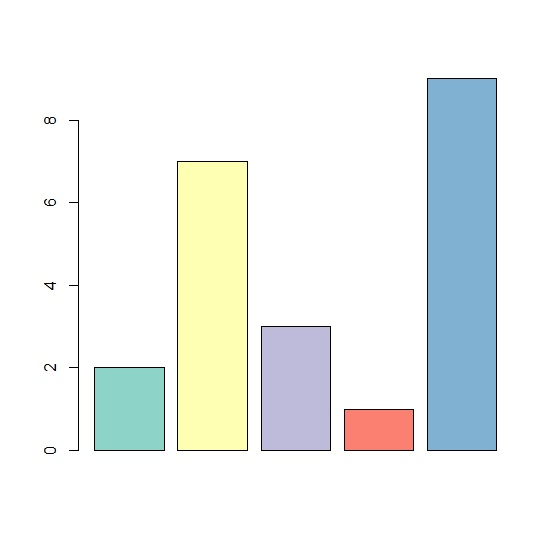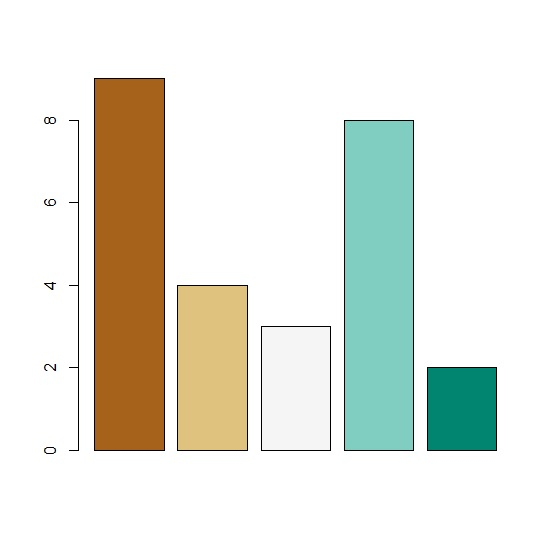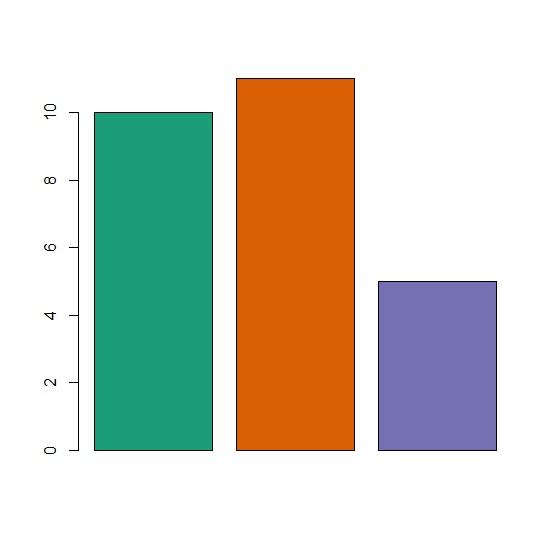# How to create a bar plot in R filled with color palette in RColorBrewer package?

To create a bar plot in R filled with color palette in RColorBrewer package, we can follow the below steps −

• First of all, create a vector.
• Create the bar plot with color palette in RColorBrewer package.

## Example 1

Let’s create a vector as shown below −

Live Demo

x<-sample(1:10,5)
x

On executing, the above script generates the below output(this output will vary on your system due to randomization) −

## Output

 2 7 3 1 9

## Create the bar plot

Using brewer.pal to color the bars in bar plot −

x<-sample(1:10,5)
barplot(x,col=brewer.pal(n=5,name="Set3"))

#### Output## Example 2

Let’s create a vector as shown below −

Live Demo

y<-sample(1:9,5)
y
 9 4 3 8 2

## Create the bar plot

Using brewer.pal to color the bars in bar plot −

y<-sample(1:9,5)
barplot(y,col=brewer.pal(n=5,name="BrBG"))

#### Output## Example 3

Let’s create a vector as shown below −

Live Demo

z<-rpois(3,10)
z
 10 11 5

## Create the bar plot

Using brewer.pal to color the bars in bar plot −

z<-rpois(3,10)
barplot(z,col=brewer.pal(n=3,name="Dark2"))

#### Output